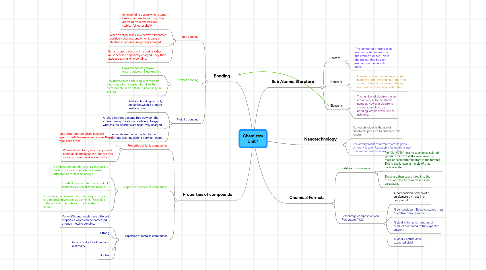# Chemistry Unit 1

Commencez. C'est gratuit
ou s'inscrire avec votre adresse courrielChemistry Unit 1 par## 1. Sub Atomic Sturcture

### 1.1. Protons

1.1.1. The number of protons in an element is determined by the atomic number. This is the top number by each element on the periodic table

### 1.2. Neutrons

1.2.1. Element's mass number determines number of protons and neutrons in an atom. This is the bottom number by each element on the periodic table.

### 1.3. Electrons

1.3.1. The number of electrons in an atom is equal to the atomic number. However electrons are transferable so by bonding, atoms often lose or gain one.

## 2. Bonding

### 2.1. Ionic bonding

2.1.1. Ionic bonding occurs when atoms lose or gain electrons from other atoms to make themselves stable. (full outer shell)

2.1.2. When an atom loses an electron it becomes positively charged, and when it gains an electron it becomes negatively charged.

2.1.3. For an atom to become charged, another must become oppositely charged. They then attract causing ionic bonding.

### 2.2. Covalent bonding

2.2.1. Covalent bonding always occurs between 2 non-metals

2.2.2. The atoms share either single or multiple electrons which causes a bond. As the electrons are shared there is no change in charge.

### 2.3. Metallic bonding

2.3.1. Metallic bonding can only occur between 2 or more metal atoms

2.3.2. All the electrons become free between the atoms making the atoms positively charged whereas the electrons are negatively charged.

2.3.3. The metals are then attracted to the electrons creating a bond between them.

## 3. Properties of compounds

### 3.1. Properties of Ionic compounds

3.1.1. Ionic structures are brittle because if 1 layer of particles are moved across the all repel each other.

3.1.2. When in liquid form, ionic compounds conduct electricity as the ions are free moving so can travel as electricity.

### 3.2. Properties of covalent compounds

3.2.1. Covalent compounds have low boiling and melting points as they are only weakly attracted as there is no charge.

3.2.2. Covalent compounds don't conduct electricity as they have no charge.

3.2.3. However some covalent bonds are very strong and hard to break down such as diamond. This is due to the amount of bonds each carbon atoms creates.

### 3.3. Properties of metallic compounds

3.3.1. Many different metals have different properties which can be harnessed through metallic bonding.

3.3.2. Strong

3.3.3. Good conductor of heat and electricity

3.3.4. Ductile

## 5. Chemical Formula

### 5.1. Relative formula mass

5.1.1. For relative formula mass to be calculated, you must first know the relative atomic mass of each different atom in the formula. This is also known as 1 mole of that particular atom.

5.1.2. These are then substituted into the formula to the formula mass can be calculated.

### 5.2. Percentage composition and Percentage Yield.

5.2.1. % composition is the % of a an element by mass in a compound

5.2.2. % composition = Relative atomic mass / Relative formula mass.

5.2.3. % yield is the actual amount of product compared to the expected amount.

5.2.4. % yield = actual yield / expected yield